Get instant live expert help with Excel or Google Sheets“My Excelchat expert helped me in less than 20 minutes, saving me what would have been 5 hours of work!”

#### Post your problem and you’ll get Expert help in seconds.

Your message must be at least 40 characters
Our professional Expert are available now. Your privacy is guaranteed.

# A Guide on Using SUMIF with the Time Format

The SUMIF function not only sums numbers but also time.  The syntax and usage is pretty much the same, but in handling data in time format, it is really important to set the format right.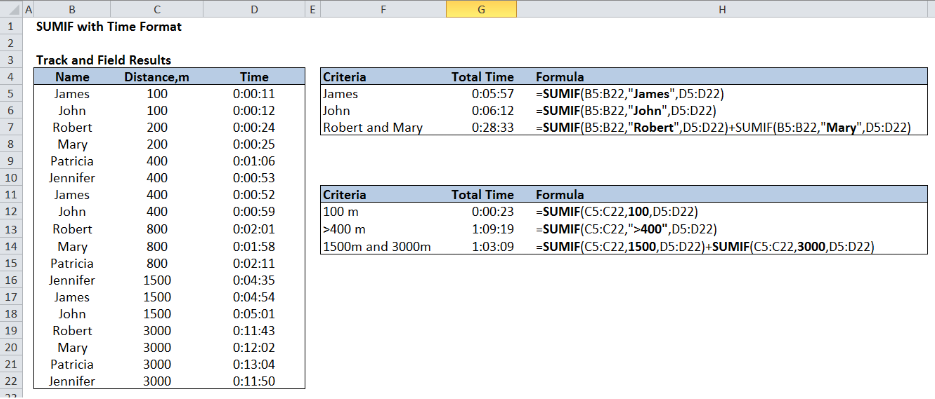Figure 1.  Using SUMIF with the time format

Formula for single criteria `=SUMIF(B5:B22,"James",D5:D22)`

Formula for multiple criteria `=SUMIF(B5:B22,"Robert",D5:D22)+SUMIF(B5:B22,"Mary",D5:D22)`

## Setting up the Data

The table below shows track and field distance and results in time format.  Note that column D for the time is in the format h:mm:ss.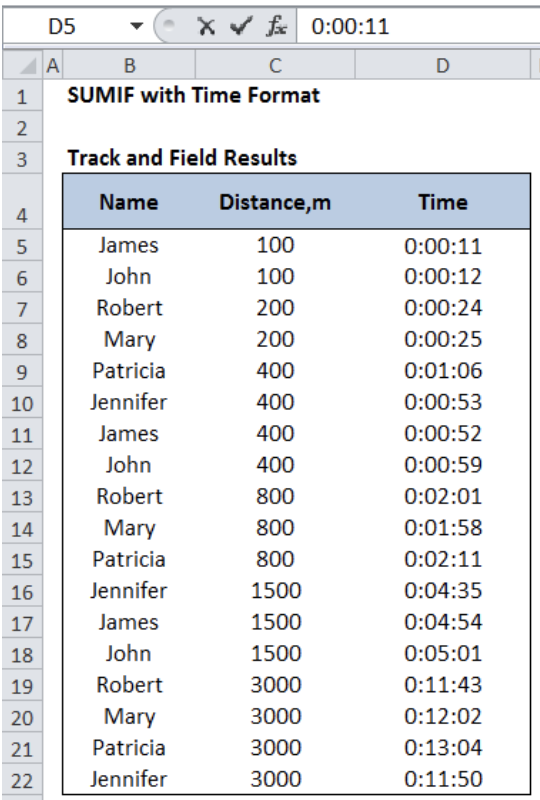Figure 2.  Sample data for SUMIF with time format

In Excel, the default format is normally set to General.  If this was the case, our data would most probably look like this: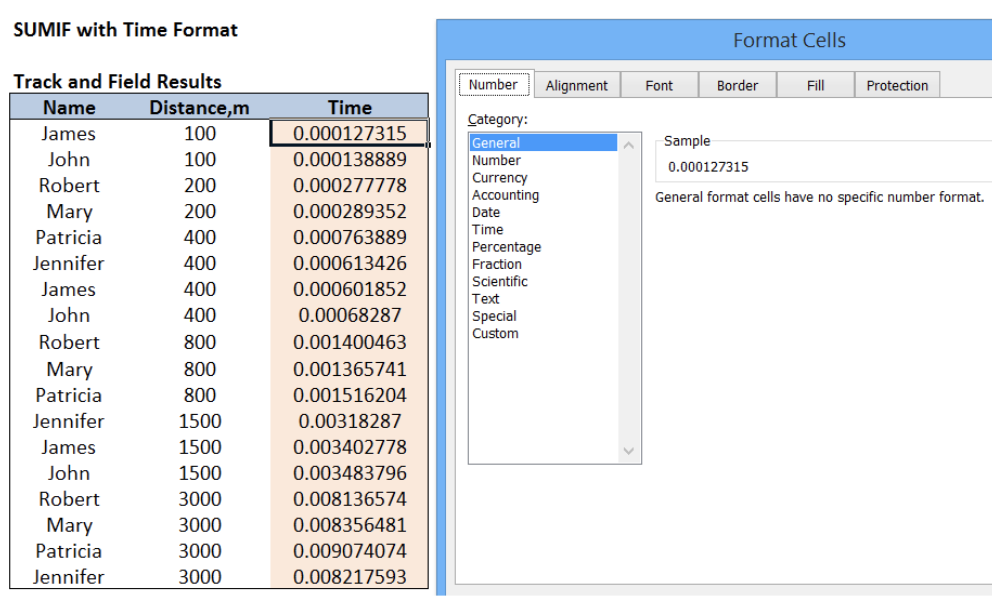Figure 3.  Sample data showing the time in General format

The time displayed in Column D is in the general format, which is the equivalent decimal value of the time entered.

## How to set the right time format?

Step 1. Select the data range for the time.  In this case, select cells D5:D22.

Step 2. Press Ctrl + 1 to launch the Format Cells dialog box.

Step 3. Select the format h:mm:ss under Custom.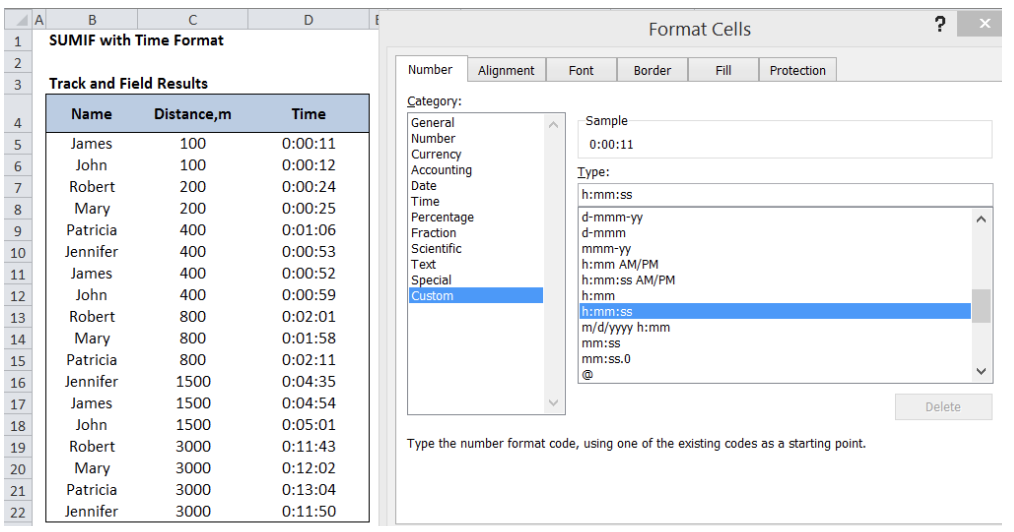Figure 4.  Setting the right time format to h:mm:ss

## Using SUMIF with the Time Format

SUMIF is a function that sums the values in a specified range, based on one criterion.

### Syntax

`=SUMIF(range,criteria, [sum_range])`

Where

• Range: the data range that will be evaluated using the criteria
• Criteria: the criteria or condition that determines which cells will be added
• Sum_range: the cells that will be added; if left blank, “sum_range” = “range” which means that the range of data that will be added is the same range of data evaluated

## SUMIF Time with a single criterion

### Example : Determine the total time for James.

In cell G5, enter the formula:

`=SUMIF(B5:B22,"James",D5:D22)`

This formula means that for all values in B5:B22 with the text “James”, the corresponding time in column D will be added.  The result is “0:05:57”.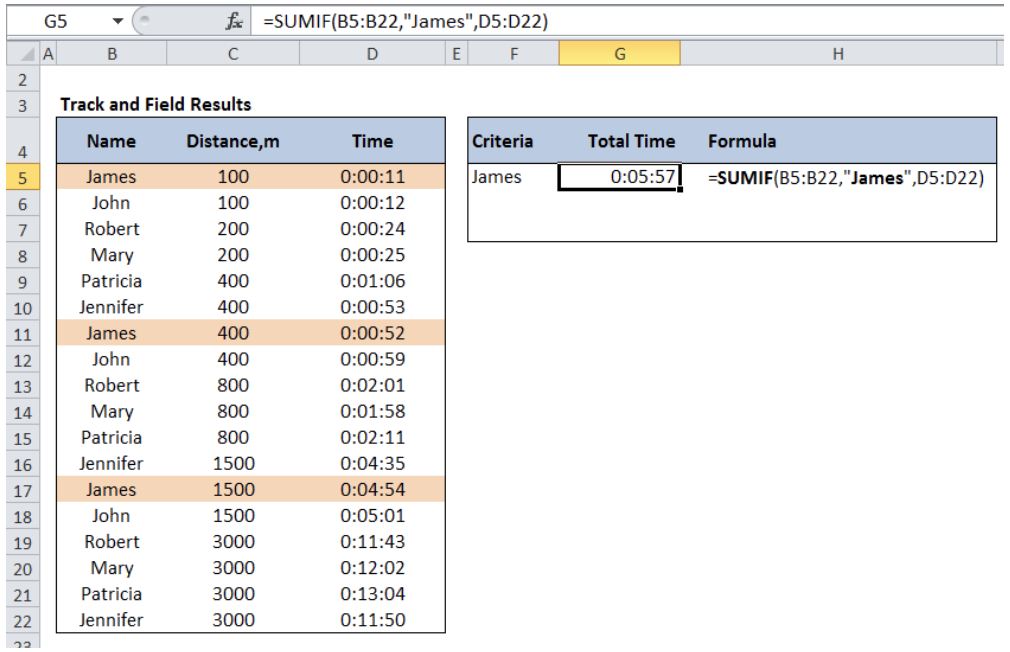Figure 5.  Entering the formula for SUMIF in time format with a single criterion

See below examples for the use of SUMIF with time format.

## SUMIF Time with multiple criteria

When we need to sum values based on multiple criteria, we add two or more SUMIF functions.

### Syntax

`=[SUMIF] + [SUMIF]+...`

`=SUMIF(range1, criteria1, [sum_range1]) + SUMIF(range2, criteria2, [sum_range2])+...`

### Example: Determine the total time for Robert and Mary.

In cell G7, enter the formula:

`=SUMIF(B5:B22,"Robert",D5:D22)+SUMIF(B5:B22,"Mary",D5:D22)`

This formula sums the time for both Robert and Mary, which is 0:28:33.

Refer to below table for other examples of SUMIF with time format in combination with different criteria.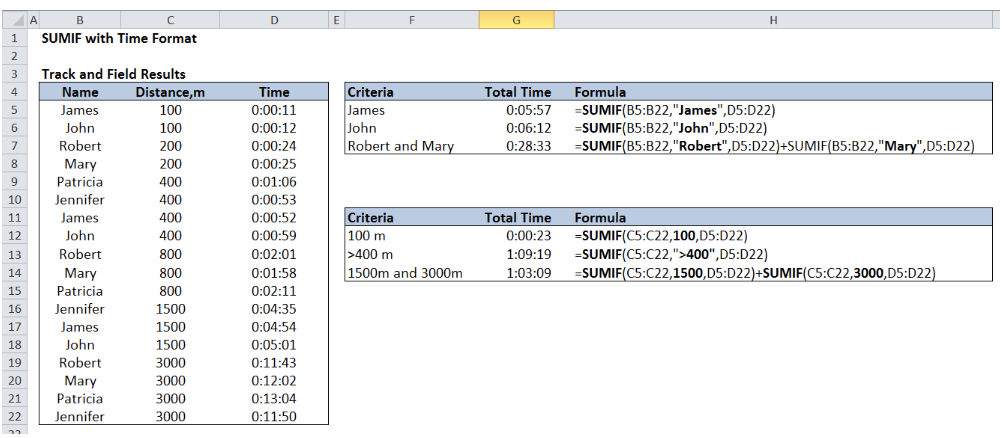Figure 6.  Output: SUMIF with time format showing different criteria and formula

### Note:

When the sum of time is greater than 24 hours, it is important to set the right format to [h]:mm:ss.

For illustration,let us consider below data and sum the hours worked by Anna using the formula:

`=SUMIF(B4:B21,"Anna",F4:F21)`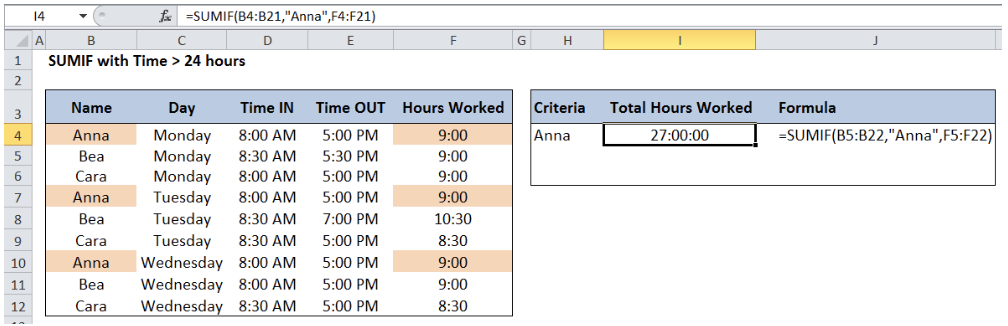Figure 7.  Example for SUMIF with time greater than 24 hours

The result is 27 hours.  However, if we have used the format h:mm:ss, we would have obtained a wrong result.  Below table shows the comparison.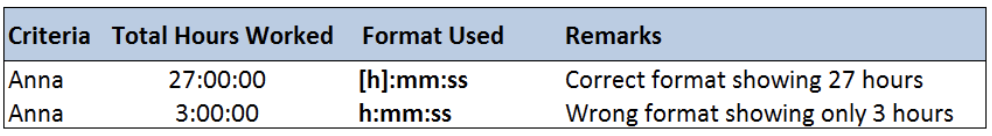Figure 8.  Comparison of SUMIF with time using different formats

Most of the time, the problem you will need to solve will be more complex than a simple application of a formula or function. If you want to save hours of research and frustration, try our live Excelchat service! Our Excel Experts are available 24/7 to answer any Excel question you may have. We guarantee a connection within 30 seconds and a customized solution within 20 minutes.

### Did this post not answer your question? Get a solution from connecting with the expert.Another blog reader asked this question today on Excelchat:
Solution examplesamending a formula so that there is another criteria and if it does not meet this criteria then we stick with the original criteria
Solved by A. B. in 40 minsI need Column D to = Column C - Column A only if Column B has a 'Date' entered.
Solved by I. B. in 11 minsHello, I need help trying to create a sum of numerical values on a spreadsheet in which the only values that apply to the sum are when the status of another cell is a certain value (e.g. value is not in sum when status: closed, value is in sum when status: open)
Solved by T. W. in 18 minsI am looking for a formula to SUM column L, if Column A matched the relevant date and column C matches the name
Solved by Z. Y. in 16 minsI need a formula that is essentially saying IF this range of cells contain a certain name, add the number in another cell on the same row, and them sum that number in a different cell..!
Solved by O. H. in 44 mins## Subscribe to Excelchat.coAnother blog reader asked this question today on Excelchat: